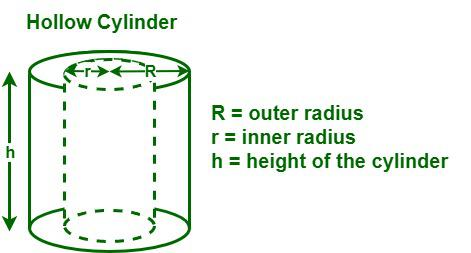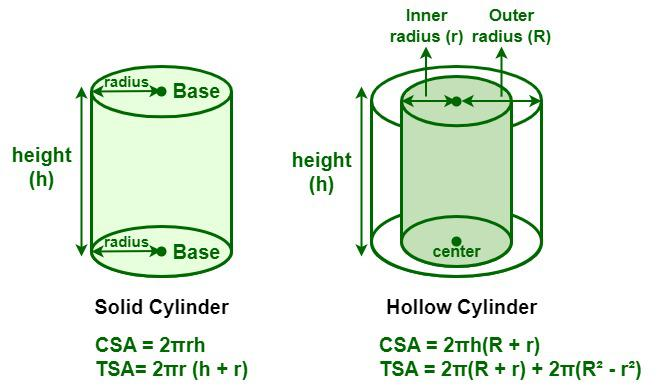GFG App
Open AppBrowser
Continue

# Area of Hollow Cylinder

A hollow cylinder is a cylinder that is hollow from the inside. A hollow cylinder is defined as a three-dimensional object that is empty from the inside. In a hollow cylinder, there are two circular bases in the shape of rings. The circular base has two radii a smaller inner radius and a bigger outer radius.

## Hollow CylinderA hollow cylinder is defined as a cylinder that is empty from the inside and has a difference between the internal and external radius. There is some thickness enclosed between the inner radius and the outer radius, of the hollow cylinder, The thickness between them is equal to the difference between the internal and external radius. The height, of the hollow cylinder, is the perpendicular distance between its two circular bases.

Some examples of Hollow cylinders are water pipes, straws, water bottles, etc.

## Area of Hollow Cylinder Formula

Finding the area of a hollow cylinder is similar to finding the area of a cylinder. A hollow cylinder has two types of surface areas, i.e., a curved surface area and a total surface area. The curved surface area, or lateral surface area, is the surface area of the curved surface of the hollow cylinder. The total surface area of the hollow cylinder is the sum of its curved surface area and the areas of its two circular bases.## Lateral Surface Area

Now, let’s calculate the curved surface area of the hollow cylinder. The curved surface area (CSA) of the hollow cylinder is equal to the sum of the external surface area (ESA) and the internal surface area (ISA) of the cylinder.

Let C1 be the outer circumference and C2 be the inner circumference of the given cylinder.

Thickness of the hollow cylinder (t) = R − r

We know that,

Circumference of a circle (C) = 2πr

So, C1 = 2πR and C2 = 2πr

CSA = ESA + ISA

We know that,

Curved Surface Area of a solid cylinder = C × h = 2πrh

CSA = 2πR × h + 2πr × h
= 2πRh + 2πrh
= 2πh (R + r)

Curved Surface Area of Hollow Cylinder = 2πh (R + r) square units

where,
h” is the height of the hollow cylinder,
R” is the outer radius of the given cylinder, and
r” is the inner radius of the given cylinder.

## Total Surface Area

Total Surface Area = Curved Surface Area + Areas of Bases

Let A1 be the area enclosed by the inner radius, r, and A2 be the area enclosed by the outer radius, R.

We know that the area of a circle (A) = πr2

So, A1 = πR2 and A2 = πr2

Now, the cross-sectional area of the base of the hollow cylinder (A) = A1 − A2

A = πR2 − πr2 = π((R² – r²)

TSA = CSA + 2 × A
= 2πh (R + r) + 2 × π(R² – r²)

TSA = 2πh (R + r) + 2π(R² – r²)

Total Surface Area of Hollow Cylinder = [2πh (R + r) + 2π(R² – r²)] square units

where,
h” is the height of the hollow cylinder,
R” is the outer radius of the given cylinder, and
r” is the inner radius of the given cylinder.

## How to Find the Area of Hollow Cylinder?

Let’s take an example to understand how to calculate the area of a hollow cylinder.

Example: Calculate the area of a hollow cylinder whose external radius is 12 cm, the internal radius is 9 cm, and the height is 7 cm

Solution:

Step 1: Note the values of the given dimensions. Here, the external radius (R) is 12 cm, the internal radius (r) is 9 cm, and the height (h) is 7 cm.

Step 2: We know that the formula to find the area (TSA) of a hollow cylinder is [2πh (R + r) + 2π(R² – r²)] square units. Now, substitute the given values in the formula.

Step 3: Thus, the area of a hollow cylinder is calculated as

TSA = 1319.469 Square Meters

## Solved Examples on Area of Hollow Cylinder

Example 1: Calculate the area of a hollow cylinder whose external radius is 8 cm, the internal radius is 4 cm, and the height is 12 cm [Use π= 22/7]

Solution:

Height = 12 cm

We know that,

Area of the hollow cylinder = 2πh (R + r) + 2π(R2 – r2)
= 2 × (22/7) × 12 × (8 + 4) + 2 × (22/7) × (82 – 42)
= 2 × (22/7) × 10 × 15 + 2 × (22/7) × 48
= 905.142 + 301.714
= 1,206.856 sq. cm.

Hence, the area of a hollow cylinder is 1,206.856 sq. cm.

Example 2: Calculate the area of a hollow cylinder whose external radius is 6 m, the internal radius is 2 m, and the height is 8 m [Use π= 22/7]

Solution:

Height = 8 m

We know that,

Area of the hollow cylinder = 2πh(R + r) + 2π(R2 – r2)
= 2 × (22/7) × 8 × (6 + 2) + 2 × (22/7) × (62 – 22)
= 2 × (22/7) × 10 × 15 + 2 × (22/7) × 32
= 402.286 + 201.143
= 603.429 sq. m.

Hence, the area of a hollow cylinder is 603.429 sq. m.

Example 3: Ram has a hollow cylindrical pipe with him, and he was asked to find its curved surface area. The external radius of the pipe is 10 inches, the internal radius is 6 inches, and the height is 14 inches. [Use π= 22/7]

Solution:

Height = 8 m

We know that,

Curved Surface Area of a Hollow cylinder = 2πh (R + r)
= 2 × (22/7) × 14 × (10 + 6)
= 2 × (22/7) × 14 × 16
= 1,408 sq. in

Hence, the curved surface area of the given hollow cylindrical pipe is 1,408 sq. in.

Example 4: Calculate the curved surface area of a hollow cylinder whose outer diameter is 26 cm, the inner diameter is 18 cm, and the height is 12 cm. [Use π= 22/7]

Solution:

Outer diameter = 26 cm

So, external Radius (R) = 26 cm/ 2 = 13 cm

Inner diameter = 18 cm

So, internal Radius (r) = 18 cm/2 = 9 cm

Height = 12 cm

We know that,

Curved Surface Area of a Hollow cylinder = 2πh (R + r)
= 2 × (22/7) × 12 × (13 + 9)
= 2 × (22/7) × 12 × 22
= 1,659.428 sq. cm

Hence, the curved surface area of the given hollow cylindrical pipe is 1,659.428 sq. cm.

## FAQs on Hollow Cylinder

Question 1: What is a hollow cylinder? Give some examples of a hollow cylinder.

A hollow cylinder can be defined as a three-dimensional geometric object that is empty from the inside. A hollow cylinder consists of two circular bases that have inner and outer radii. Straws, water pipes, tubes, toilet paper rolls, etc. are some examples of hollow cylinders that we see in our daily lives.

Question 2: What is the formula to calculate the thickness of a hollow cylinder?

The thickness of a hollow cylinder is the enclosed space between the inner radius and the outer radius, which is equal to the difference between the internal and external radius.

Thickness of the hollow cylinder (t) = R − r

where,
R” is the outer radius of the given cylinder, and
r” is the inner radius of the given cylinder.

Question 3: What is the formula to calculate the curved surface area of a hollow cylinder?

The curved surface area, or lateral surface area, is the surface area of the curved surface of a hollow cylinder that is equal to the sum of the external surface area (ESA) and the internal surface area (ISA) of the cylinder.

Curved Surface Area of Hollow Cylinder = 2πh (R + r) square units

where,
h” is the height of the hollow cylinder,
R” is the outer radius of the given cylinder, and
r” is the inner radius of the given cylinder.

Question 4: What is meant by the annular ring of a hollow cylinder?

The circular bases of a hollow cylinder are similar to an annular ring, which is a region bounded by two concentric circles. So, the area of the circular base of the hollow cylinder is equal to the area of the annular ring of the cylinder.

Question 5: What is the formula to calculate the volume of a hollow cylinder?

The formula to calculate the volume of a hollow cylinder is given as follows:

Volume of Hollow Cylinder = π(R2 -r2)h cubic units

where,
h” is the height of the hollow cylinder,
R” is the outer radius of the given cylinder, and
r” is the inner radius of the given cylinder.

### Related Resource

My Personal Notes arrow_drop_up